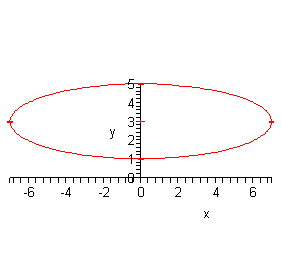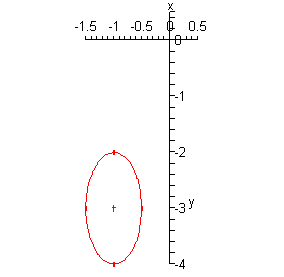Paul's Online Notes
Home / Algebra / Common Graphs / Ellipses
Show Mobile Notice Show All Notes Hide All Notes
Mobile Notice
You appear to be on a device with a "narrow" screen width (i.e. you are probably on a mobile phone). Due to the nature of the mathematics on this site it is best views in landscape mode. If your device is not in landscape mode many of the equations will run off the side of your device (should be able to scroll to see them) and some of the menu items will be cut off due to the narrow screen width.

### Section 4.3 : Ellipses

In a previous section we looked at graphing circles and since circles are really special cases of ellipses we’ve already got most of the tools under our belts to graph ellipses. All that we really need here to get us started is then standard form of the ellipse and a little information on how to interpret it.

Here is the standard form of an ellipse.

$\frac{{{{\left( {x - h} \right)}^2}}}{{{a^2}}} + \frac{{{{\left( {y - k} \right)}^2}}}{{{b^2}}} = 1$

Note that the right side MUST be a 1 in order to be in standard form. The point $$\left( {h,k} \right)$$ is called the center of the ellipse.

To graph the ellipse all that we need are the right most, left most, top most and bottom most points. Once we have those we can sketch in the ellipse. Here are formulas for finding these points.

\begin{align*} & {\mbox{right most point : }}\left( {h + a,k} \right)\\ & {\mbox{left most point : }}\left( {h - a,k} \right)\\ & {\mbox{top most point : }}\left( {h,k + b} \right)\\ & {\mbox{bottom most point : }}\left( {h,k - b} \right)\end{align*}

Note that a is the square root of the number under the x term and is the amount that we move right and left from the center. Also, b is the square root of the number under the y term and is the amount that we move up or down from the center.

Let’s sketch some graphs.

Example 1 Sketch the graph of each of the following ellipses.
1. $$\displaystyle \frac{{{{\left( {x + 2} \right)}^2}}}{9} + \frac{{{{\left( {y - 4} \right)}^2}}}{{25}} = 1$$
2. $$\displaystyle \frac{{{x^2}}}{{49}} + \frac{{{{\left( {y - 3} \right)}^2}}}{4} = 1$$
3. $$4{\left( {x + 1} \right)^2} + {\left( {y + 3} \right)^2} = 1$$
Show All Solutions Hide All Solutions
a $$\displaystyle \frac{{{{\left( {x + 2} \right)}^2}}}{9} + \frac{{{{\left( {y - 4} \right)}^2}}}{{25}} = 1$$ Show Solution

So, the center of this ellipse is $$\left( { - 2,4} \right)$$ and as usual be careful with signs here! Also, we have $$a = 3$$ and $$b = 5$$. So, the points are,

\begin{align*} & {\mbox{right most point : }}\left( {1,4} \right)\\ & {\mbox{left most point : }}\left( { - 5,4} \right)\\ & {\mbox{top most point : }}\left( { - 2,9} \right)\\ & {\mbox{bottom most point : }}\left( { - 2, - 1} \right)\end{align*}

Here is a sketch of this ellipse.b $$\displaystyle \frac{{{x^2}}}{{49}} + \frac{{{{\left( {y - 3} \right)}^2}}}{4} = 1$$ Show Solution

The center for this part is $$\left( {0,3} \right)$$ and we have $$a = 7$$ and $$b = 2$$. The points we need are,

\begin{align*}& {\mbox{right most point : }}\left( {7,3} \right)\\ & {\mbox{left most point : }}\left( { - 7,3} \right)\\ & {\mbox{top most point : }}\left( {0,5} \right)\\ & {\mbox{bottom most point : }}\left( {0,1} \right)\end{align*}

Here is the sketch of this ellipse.c $$4{\left( {x + 1} \right)^2} + {\left( {y + 3} \right)^2} = 1$$ Show Solution

Now with this ellipse we’re going to have to be a little careful as it isn’t quite in standard form yet. Here is the standard form for this ellipse.

$\frac{{{{\left( {x + 1} \right)}^2}}}{{\frac{1}{4}}} + {\left( {y + 3} \right)^2} = 1$

Note that in order to get the coefficient of 4 in the numerator of the first term we will need to have a $$\frac{1}{4}$$ in the denominator. Also, note that we don’t even have a fraction for the y term. This implies that there is in fact a 1 in the denominator. We could put this in if it would be helpful to see what is going on here.

$\frac{{{{\left( {x + 1} \right)}^2}}}{{\frac{1}{4}}} + \frac{{{{\left( {y + 3} \right)}^2}}}{1} = 1$

So, in this form we can see that the center is $$\left( { - 1, - 3} \right)$$ and that $$a = \frac{1}{2}$$ and $$b = 1$$. The points for this ellipse are,

\begin{align*} & {\mbox{right most point : }}\left( { - \frac{1}{2}, - 3} \right)\\ & {\mbox{left most point : }}\left( { - \frac{3}{2}, - 3} \right)\\ & {\mbox{top most point : }}\left( { - 1, - 2} \right)\\ & {\mbox{bottom most point : }}\left( { - 1, - 4} \right)\end{align*}

Here is this ellipse.Finally, let’s address a comment made at the start of this section. We said that circles are really nothing more than a special case of an ellipse. To see this let’s assume that $$a = b$$. In this case we have,

$\frac{{{{\left( {x - h} \right)}^2}}}{{{a^2}}} + \frac{{{{\left( {y - k} \right)}^2}}}{{{a^2}}} = 1$

Note that we acknowledged that $$a = b$$ and used a in both cases. Now if we clear denominators we get,

${\left( {x - h} \right)^2} + {\left( {y - k} \right)^2} = {a^2}$

This is the standard form of a circle with center $$\left( {h,k} \right)$$ and radius a. So, circles really are special cases of ellipses.